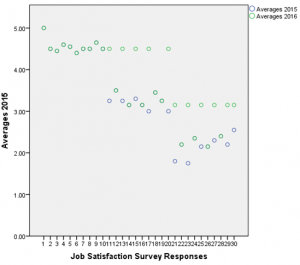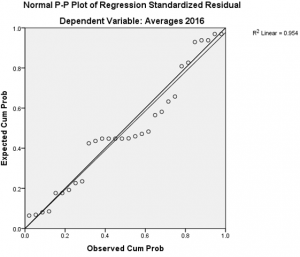# SPSS Task Harvard Case Solution & Analysis

Figure 1.2: Scatter plot

#Linear Regression:

Research Question:

Does average job satisfaction level of the year 2015 is a predictor of average job satisfaction level of the year 2016?

Null Hypothesis: Average job satisfaction level of the year 2015 is not a predictor of average job satisfaction level of the year 2016.

Alternate Hypothesis: Average job satisfaction level of the year 2015 is a predictor of average job satisfaction level of the year 2016.

Regression Equation:

Averages 2016            =          1.449 + 0.693 (Averages 2015)

On the basis of p value < 0.001 at 95% confidence level, there has been found the strong statistically significant evidences to reject null hypothesis that states average job satisfaction level of the year 2015 is not a predictor of average job satisfaction level of the year 2016. By accepting the alternative hypothesis that the average job satisfaction level of the year 2015 is a predictor of average job satisfaction level of the year 2016, it has been found that R square is 0.653 that indicates 65.3% data variability around its mean. Coefficient of independent variable 0.693 predict that when a year increases, job satisfaction level increases by 0.693 points while R square shows the accuracy of this prediction which is 65.3%.

### Table 1.4: Linear Regression

 Model Summary Model R R Square Adjusted R Square Std. Error of the Estimate 1 .808a .653 .640 .51926 a. Predictors: (Constant), Averages 2015 b. Dependent Variable: Averages 2016

 ANOVA Model Sum of Squares df Mean Square F Sig. 1 Regression 14.187 1 14.187 52.618 .000 b Residual 7.550 28 .270 Total 21.737 29 a. Dependent Variable: Averages 2016 b. Predictors: (Constant), Averages 2015

 Coefficients Model Unstandardized Coefficients Standardized Coefficients t Sig. 95.0% Confidence Interval for B B Std. Error Beta Lower Bound Upper Bound 1 (Constant) 1.449 .332 4.368 .000 .769 2.128 Averages 2015 .693 .096 .808 7.254 .000 .497 .889 a. Dependent Variable: Averages 2016

Figure 1.3: Normal P-P Plot

#One Way Anova:

Research Question:

Is there a significant mean value differences among all three groups in the years 2015 and 2016?

Null Hypothesis: A mean value is same among all three groups in the years 2015 and 2016.

Alternate Hypothesis:A mean value is not same among all three groups in the year 2015 and 2016.

On the basis of p value < 0.001 at 95% confidence level, there has been found the strong statistically significant evidences to reject null hypothesis that states a mean value is same among all three groups in the years 2015 and 2016. By accepting the alternative hypothesis that a mean value is not same among all three groups in the year 2015 and 2016, it has been found that a mean value for three groups in the year 2015 are 4.565, 3.23 and 2.185 respectively while a mean value for three groups in the year 2016 are 4.565, 3.9 and 2.8 respectively.

### Table 1.5: One Way Anova

 Descriptive N Mean Std. Deviation Std. Error 95% Confidence Interval for Mean Minimum Maximum Lower Bound Upper Bound Averages 2015 1 10 4.5650 .16841 .05326 4.4445 4.6855 4.40 5.00 2 10 3.2300 .16533 .05228 3.1117 3.3483 3.00 3.50 3 10 2.1850 .24950 .07890 2.0065 2.3635 1.75 2.55 Total 30 3.3267 1.00899 .18422 2.9499 3.7034 1.75 5.00 Averages 2016 1 10 4.5650 .16841 .05326 4.4445 4.6855 4.40 5.00 2 10 3.9000 .64205 .20303 3.4407 4.3593 3.15 4.50 3 10 2.8000 .45704 .14453 2.4731 3.1269 2.15 3.15 Total 30 3.7550 .86576 .15807 3.4317 4.0783 2.15 5.00

 ANOVA Sum of Squares df Mean Square F Sig. Averages 2015 Between Groups 28.462 2 14.231 361.978 .000 Within Groups 1.061 27 .039 Total 29.524 29 Averages 2016 Between Groups 15.892 2 7.946 36.702 .000 Within Groups 5.845 27 .216 Total 21.737 29

Post Hoc (Tukey) Tests shows the multiple comparisons of all groups for both of the years and indicates that there is a significant mean differences among all groups at 0.05 significance level. By comparison of group means from 2015 to 2016, group 1 shows no difference in the mean from 2015 job satisfaction level to 2016 job satisfaction level while there has been found a difference between two years group means for group 2 and 3 as it can be seen in figure 1.4.

### Table 1.6: Post Ho c Tests

 Dependent Variable (I) Job Satisfaction Survey Responses (J) Job Satisfaction Survey Responses Mean Difference (I-J) Std. Error Sig. 95% Confidence Interval Lower Bound Upper Bound Averages 2015 1 2 1.33500* .08867 .000 1.1151 1.5549 3 2.38000* .08867 .000 2.1601 2.5999 2 1 -1.33500* .08867 .000 -1.5549 -1.1151 3 1.04500* .08867 .000 .8251 1.2649 3 1 -2.38000* .08867 .000 -2.5999 -2.1601 2 -1.04500* .08867 .000 -1.2649 -.8251 Averages 2016 1 2 .66500* .20808 .010 .1491 1.1809 3 1.76500* .20808 .000 1.2491 2.2809 2 1 -.66500* .20808 .010 -1.1809 -.1491 3 1.10000* .20808 .000 .5841 1.6159 3 1 -1.76500* .20808 .000 -2.2809 -1.2491 2 -1.10000* .20808 .000 -1.6159 -.5841 *. The mean difference is significant at the 0.05 level.

Figure 1.4: Bar ChartThis is just a sample partical work. Please place the order on the website to get your own originally done case solution.

### Search Case Solutions